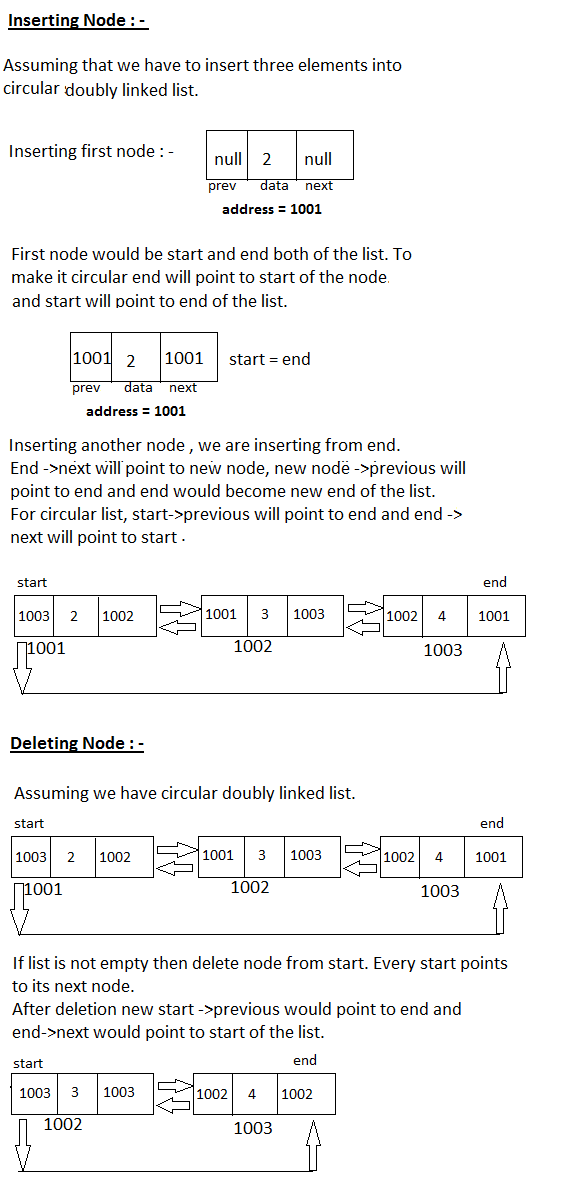as the name suggest a list that nodes are connecting in circle form. Circular Linked List is actually circular form of Singly or Doubly linked list.

Singly or Doubly list operations implementation would be same as per list's behavior, we will maintain circular connection between the start and end nodes to make it as circular.

In "Singly Linked List" every start, points to its next node and so on, end node of list does not pointing to any node so that next part contains NULL.

To make it Circular List - end node will point to start node of the list.
Click on the link -  Singly Linked List concepts and it's implementation

Same as in "Doubly Linked List" every node pointing its next and previous nodes, start node's previous does not point any node and
end node's next does not point any node both holds NULL

To make it circular start ->previous will point end node and end ->next will point to start of the list.
Click on the link - Doubly Linked List concepts and it's implementation

It makes traversing fast and such type of list use in games, time-sharing operations of operating systems etc.

Circular Linked List Operations - Implementation

Here I will explain circular list operations with both Singly and Doubly linked list. You can learn how to insert, delete nodes, and traverse the circular list.

We can insert and delete nodes in three different ways.

Insertion/deletion from start, end and at any index, to understand circular concept here i will explain insertion from end and deletion from start, which is basic or standard form of list.

If you want to checkout other ways for insertion and deletion please go through with the links that I have mentioned above in the page.

Circular Singly Linked List  : -

Insertion Process : -

Assume start and end of the list is null, i.e. there is no node element available in the list.

With every insertion node will create with data value, new node is not pointing to any node so that initially next of node will be set as null.

``````
start = null;

end = null;

node = create_node();  // {data= value , next = null }```      ```
If list is empty then this new would become start and end of the list.
``````

if ( start == null ) {

start = node;

end = node;

}```      ```
If list is not empty, insert new node from end of the list.

Existing end will point to new node and new node would become new end
node of the list.
``````
else {

end ->next = node;

node =  end;

}```    ```
Now will make it circular list, every end node will point to start of the list.

Deletion Process : -

For every deletion first make sure that list is not empty.

``````
if ( start == null ) {

print "Cannot delete node, List is empty ";

}```   ```
If list is not empty, we are deleting nodes from start.

Start -> next would become new start and end node's ->next will have new start address.

``````
else {

start = start -> next;

end ->next = start;

}
``````Circular Singly Linked List - Inserting and Deleting Node. ByTechAchievers.com

Circular Doubly Linked List  : -

Insertion Process : -

Assume start and end of the list is null, i.e. there is no node element available
in the list.

Doubly Linked List node points two different nodes, first its previous node
and second is its next node.

With every insertion node will create with data value and initially there is no other node available so that node previous and next both pointers will be set
as null.
``````
start = null;

end = null;

node = create_node(); // {data= value, previous = null, next = null }
``````
If list is empty then this new would become start and end of the list.
``````

if ( start == null ) {

start = node;

end = node;

}``````
If list is not empty, insert new node from end of the list.

Existing end ->next will point to new node and new node's -> previous will point to end after that new node would become new end of the list.
``````
else {

end ->next = node;

node->previous =  end;

end = node;

}
``````
Now will make circular list, every end node ->next will point to start and start->previous will point to end.
``````
end -> next = start;

start ->previous = end;

``````

Deletion Process : -

For every deletion first make sure that list is not empty.
``````
if ( start == null ) {

print "Cannot delete node, List is empty ";

}
``````
If list is not empty, we are deleting nodes from start.

Start -> next would become new start.
End ->next will point to new start and new start ->previous will point to end of
the list.
``````
else {

start = start -> next;

end ->next = start;

start ->previous = end;

}
``````Circular Doubly Linked List - Inserting and Deleting Node.ByTechAchievers.com

Circular Linked List Implementation - Java Example

Please go thorough with the Singly Linked List and Doubly Linked List topics for Java examples.

For circular behavior start will point end node and end node will point start node of the list except that all over the implementation would remain same for both.

Click on the link -  Singly Linked List concepts and it's implementation

Click on the link - Doubly Linked List concepts and it's implementation